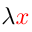Higher-Order Logic Explorer < Previous   Next > Nearby theorems Mirrors  >  Home  >  HOLE Home  >  Th. List  >  ax-inst GIF version

Axiom ax-inst 103
 Description: Instantiate a theorem with a new term. The second and third hypotheses are the HOL equivalent of set.mm "effectively not free in" predicate (see set.mm's ax-17, or ax17 205).
Hypotheses
Ref Expression
ax-inst.1 RA
ax-inst.2 ⊤⊧[(λx:α By:α) = B]
ax-inst.3 ⊤⊧[(λx:α Sy:α) = S]
ax-inst.4 [x:α = C]⊧[A = B]
ax-inst.5 [x:α = C]⊧[R = S]
Assertion
Ref Expression
ax-inst SB
Distinct variable groups:   x,y   y,B   y,S

Detailed syntax breakdown of Axiom ax-inst
StepHypRef Expression
1 ts . 2 term S
2 tb . 2 term B
31, 2wffMMJ2 11 1 wff SB
 Colors of variables: type var term This axiom is referenced by:  insti  104  leqf  169  ax9  199
 Copyright terms: Public domain W3C validator